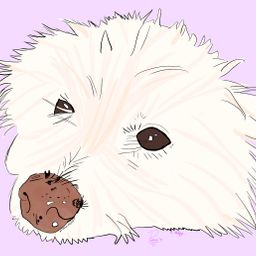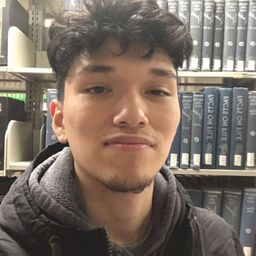🚨 Hurry, space in our FREE summer bootcamps is running out. 🚨Claim your spot here.

# Calculus with Applications

## Educators### Problem 1

Use integration by parts to find the integrals in Exercises 1-10.
$$\int 5 x e^{8 x} d x$$Julian W.
Numerade Educator

### Problem 2

Use integration by parts to find the integrals in Exercises 1–10.
$$\int 6 x e^{7 x} d x$$Bobby B.
University of North Texas

### Problem 3

Use integration by parts to find the integrals in Exercises 1–10.
$$\int(4 x-12) e^{-8 x} d x$$

Check back soon!

### Problem 4

Use integration by parts to find the integrals in Exercises 1–10.
$$\int(6 x+3) e^{-2 x} d x$$Bobby B.
University of North Texas

### Problem 5

Use integration by parts to find the integrals in Exercises 1–10.
$$\int 8 x \ln (2 x) d x$$Julian W.
Numerade Educator

### Problem 6

Use integration by parts to find the integrals in Exercises 1–10.
$$\int 6 x \ln (7 x) d x$$Bobby B.
University of North Texas

### Problem 7

Use integration by parts to find the integrals in Exercises 1–10.
$$\int_{0}^{1} \frac{6 x+5}{e^{x}} d x$$Paula M.
Numerade Educator

### Problem 8

Use integration by parts to find the integrals in Exercises 1–10.
$$\int_{0}^{1} \frac{3 x+7}{e^{x}} d x$$Bobby B.
University of North Texas

### Problem 9

Use integration by parts to find the integrals in Exercises 1–10.
$$\int_{1}^{9} \ln 3 x d x$$Julian W.
Numerade Educator

### Problem 10

Use integration by parts to find the integrals in Exercises 1–10.
$$\int_{1}^{2} \ln 5 x d x$$Bobby B.
University of North Texas

### Problem 11

Find the area between $y=(x-2) e^{x}$ and the $x$ -axis from $x=2$ to $x=4 .$Julian W.
Numerade Educator

### Problem 12

Find the area between $y=(x+1) \ln x$ and the $x$ -axis from $x=1$ to $x=e$ .Bobby B.
University of North Texas

### Problem 13

Exercises 13-22 are mixed-some require integration by parts, while others can be integrated by using techniques discussed in the chapter on Integration
$$\int x^{2} e^{8 x} d x$$William C.
Numerade Educator

### Problem 14

Exercises 13-22 are mixed-some require integration by parts, while others can be integrated by using techniques discussed in the chapter on Integration
$$\int x^{2} e^{5 x} d x$$Bobby B.
University of North Texas

### Problem 15

Exercises 13-22 are mixed-some require integration by parts, while others can be integrated by using techniques discussed in the chapter on Integration
$$\int x^{2} \sqrt{x+4} d x$$Julian W.
Numerade Educator

### Problem 16

Exercises 13-22 are mixed-some require integration by parts, while others can be integrated by using techniques discussed in the chapter on Integration
$$\int(2 x-1) \ln (3 x) d x$$Bobby B.
University of North Texas

### Problem 17

Exercises 13-22 are mixed-some require integration by parts, while others can be integrated by using techniques discussed in the chapter on Integration
$$\int(8 x+10) \ln (5 x) d x$$Julian W.
Numerade Educator

### Problem 18

Exercises 13-22 are mixed-some require integration by parts, while others can be integrated by using techniques discussed in the chapter on Integration
$$\int x^{3} e^{x^{4}} d x$$Bobby B.
University of North Texas

### Problem 19

Exercises 13-22 are mixed-some require integration by parts, while others can be integrated by using techniques discussed in the chapter on Integration
$$\int_{1}^{2}\left(1-x^{2}\right) e^{2 x} d x$$Julian W.
Numerade Educator

### Problem 20

Exercises 13-22 are mixed-some require integration by parts, while others can be integrated by using techniques discussed in the chapter on Integration
$$\int_{0}^{1} \frac{x^{2} d x}{2 x^{3}+1}$$Bobby B.
University of North Texas

### Problem 21

Exercises 13-22 are mixed-some require integration by parts, while others can be integrated by using techniques discussed in the chapter on Integration
$$\int_{0}^{1} \frac{x^{3} d x}{\sqrt{3+x^{2}}}$$Julian W.
Numerade Educator

### Problem 22

Exercises 13-22 are mixed-some require integration by parts, while others can be integrated by using techniques discussed in the chapter on Integration
$$\int_{0}^{5} x \sqrt{x^{2}+2} d x$$Bobby B.
University of North Texas

### Problem 23

Use the table of integrals, or a computer or calculator with symbolic integration capabilities, to find each indefinite integral.

$$\int \frac{16}{\sqrt{x^{2}+16}} d x$$

Check back soon!

### Problem 24

$$\int \frac{10}{x^{2}-25} d x$$Bobby B.
University of North Texas

### Problem 25

$$\int \frac{3}{x \sqrt{121-x^{2}}} d x$$Julian W.
Numerade Educator

### Problem 26

$$\int \frac{2}{3 x(3 x-5)} d x$$Bobby B.
University of North Texas

### Problem 27

$$\int \frac{-6}{x(4 x+6)^{2}} d x$$Julian W.
Numerade Educator

### Problem 28

$$\int \sqrt{x^{2}+15} d x$$Bobby B.
University of North Texas

### Problem 29

What rule of differentiation is related to integration by parts?Julian W.
Numerade Educator

### Problem 30

Explain why the two methods of solving Example 2 are
equivalent.Bobby B.
University of North Texas

### Problem 31

Suppose that $u$ and $v$ are differentiable functions of $x$ with $\int_{0}^{1} v d u=4$ and the following functional values.
$$\begin{array}{ccc}{x} & {u(x)} & {v(x)} \\ \hline 0 & {2} & {1} \\ \hline 1 & {3} & {-4}\end{array}$$
Use this information to determine $$\int_{0}^{1} u d v$$Julian W.
Numerade Educator

### Problem 32

Suppose that $u$ and $v$ are differentiable functions of $x$ with $\int_{1}^{20} v d u=-1$ and the following functional values.
$$\begin{array}{ccc}{x} & {u(x)} & {v(x)} \\ \hline 1 & {5} & {-2} \\ \hline 20 & {15} & {6}\end{array}$$
Use this information to determine $$\int_{1}^{20} u d v$$Bobby B.
University of North Texas

### Problem 33

Suppose we know that the functions $r$ and $s$ are everywheredifferentiable and that $r(0)=0 .$ Suppose we also know thatfor $0 \leq x \leq 2,$ the area between the $x$ -axis and the nonnegative function $h(x)=s(x) \frac{d r}{d x}$ is $5,$ and that on the same interval, the area between the $x$ -axis and the nonnegative function $k(x)=r(x) \frac{d s}{d x}$ is $10 .$ Determine $r(2) s(2)$Robert D.
Numerade Educator

### Problem 34

Suppose we know that the functions $u$ and $v$ are everywhere differentiable and that $u(3)=0 .$ Suppose we also know thatfor $1 \leq x \leq 3,$ the area between the $x$ -axis and the nonnegative function $h(x)=u(x) \frac{d v}{d x}$ is $15,$ and that on the same interval, the area between the $x$ -axis and the nonnegative function $k(x)=v(x) \frac{d u}{d x}$ is $20 .$ Determine $u(1) v(1)$Bobby B.
University of North Texas

### Problem 35

Use integration by parts to derive the following formula from the table of integrals.
$$\int x^{n} \cdot \ln |x| d x=x^{n+1}\left[\frac{\ln |x|}{n+1}-\frac{1}{(n+1)^{2}}\right]+C, \quad n \neq-1$$Julian W.
Numerade Educator

### Problem 36

Use integration by parts to derive the following formula from the table of integrals.
$$\int x^{n} e^{a x} d x=\frac{x^{n} e^{a x}}{a}-\frac{n}{a} \int x^{n-1} e^{a x} d x+C, \quad a \neq 0$$Bobby B.
University of North Texas

### Problem 37

(a) One way to integrate $\int x \sqrt{x+1} d x$ is to use integration by parts. Do so to find the antiderivative.
(b) Another way to evaluate the integral in part (a) is by using the substitution $u=x+1 .$ Do so to find the antiderivative.
(c) Compare the results from the two methods. If they do not look the same, explain how this can happen. Discuss the advantages and disadvantages of each method.

Check back soon!

### Problem 38

Using integration by parts,

\begin{aligned} \int \frac{1}{x} d x &=\int \frac{1}{x} \cdot 1 d x \\ &=\frac{1}{x} \cdot x-\int\left(-\frac{1}{x^{2}}\right) x d x \\ &=1+\int \frac{1}{x} d x \end{aligned}

Subtracting $\int \frac{1}{x} d x$ from both sides we conclude that $0=1 .$ What is wrong with this logic? Source: Sam Northshield.Bobby B.
University of North Texas

### Problem 39

Rate of Change of Revenue The rate of change of revenue (in dollars per calculator) from the sale of x calculators is
$$R^{\prime}(x)=(x+1) \ln (x+1)$$
Find the total revenue from the sale of the first 12 calculators. (Hint: In this exercise, it simplifies matters to write an anti derivative of $x+1$ as $(x+1)^{2} / 2$ rather than $x^{2} / 2+x$ . $)$

Check back soon!

### Problem 40

Reaction to a Drug The rate of reaction to a drug is given by
$$r^{\prime}(t)=2 t^{2} e^{-t}$$
where $t$ is the number of hours since the drug was administered. Find the total reaction to the drug from $t=1$ to $t=6 .$Bobby B.
University of North Texas

### Problem 41

Growth of a Population The rate of growth of a microbem population is given by
$$m^{\prime}(t)=27 t e^{3 t}$$
where $t$ is time in days. What is the total accumulated growthduring the first 2 days?Julian W.
Numerade Educator

### Problem 42

APPLY IT Rate of Growth The area covered by a patch of moss is growing at a rate of

$$A^{\prime}(t)=\sqrt{t} \ln t$$

$\mathrm{cm}^{2}$ per day, for $t \geq 1 .$ Find the additional amount of area covered by the moss between 4 and 9 days.Bobby B.
University of North Texas

### Problem 43

Thermic Effect of Food As we saw in an earlier chapter, a person's metabolic rate tends to go up after eating a meal and then, after some time has passed, it returns to a resting metabolic rate. This phenomenon is known as the thermic effect of food, and the effect (in kJ per hour) for one individual is
$$F(t)=-10.28+175.9 t e^{-t / 1.3}$$
where $t$ is the number of hours that have elapsed since eating a meal. Find the total thermic energy of a meal for the next six hours after a meal by integrating the thermic effect function between $t=0$ and $t=6 .$ Source: American Journal of Clinical Nutrition.Julian W.
Numerade Educator

### Problem 44

Rumen Fermentation The rumen is the first division of the stomach of a ruminant, or cud-chewing animal. An article on the rumen microbial system reports that the fraction of the soluble material passing from the rumen without being fermented during the first hour after its ingestion could be calculated by the integral
$$\int_{0}^{1} k e^{-k t}(1-t) d t$$
where $k$ measures the rate that the material is fermented. Source: Annual Review of Ecology and Systematics.
(a) Determine the above integral, and evaluate it for the following values of $k$ used in the article: $1 / 12,1 / 24,$ and 1$/ 48$ hour.
(b) The fraction of intermediate material left in the rumen at
1 hour that escapes digestion by passage between 1 and 6 hours is given by
$$\int_{1}^{6} k e^{-k t}(6-t) / 5 d t$$
Determine this integral, and evaluate it for the values of k
given in part (a).Bobby B.
University of North Texas